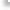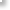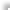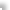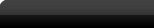HomeAbout UsSolutionsTrainingSupportFAQCustomer Service QuestionsTechnical SupportTroubleshootingKnowledge Base DownloadsContact UsSitemap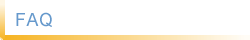Knowledge Base

 1 What is Young's Modulus (E)?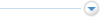Young's Modulus (E) is a measure of the stiffness of any material. It is also known as the Young Modulus, Modulus of Elasticity (MOE), Elastic Modulus or Tensile Modulus and is defined as the ratio of the uniaxial linear Stress over the uniaxial linear Strain in the range of stress in which Hooke's Law holds. This can be experimentally determined from the slope of a stress-strain curve created during destructive tensile tests conducted on a sample of the material. DEPA V9 determines the Young.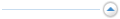2 What are the Units of Measurement of Young's Modulus?Young's Modulus (E) is defined as the ratio of Stress over Strain. Stress has the same units of Pressure and Strain is Dimensionless. So Young's Modulus has the same units as Pressure. The SI unit for Young's Modulus is the pascal (Pa or N/m²), the practical units are megapascals (MPa or N/mm²) or gigapascals (GPa or kN/mm²). In United States it is expressed as pounds (force) per square inch (psi or lb/in²). The practical units are Kpsi (Kilo psi) or Mpsi (Mega psi).1Download your DEPA V9 30 day Free Trial